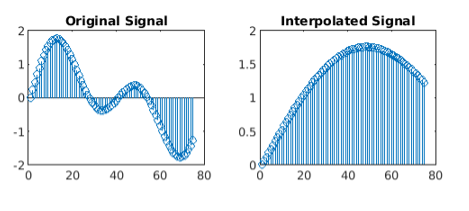Related Articles

# Up-sampling in MATLAB

• Last Updated : 01 Oct, 2020

Interpolation or up-sampling is the specific inverse of decimation. It is a data saving operation, in that all examples of x[n] are available in the extended signal y[n]. Interpolation works by adding (L–1) zero-valued examples for each input sample.

We will be using the interp() function to interpolate a signal. It is used to increase the sample rate of a signal by an integer factor.

Syntax: a = interp(x, r)
Parameter:

• x: input signal
• r: interpolation factor

Return Value: Returns interpolated signal

MATLAB code for interpolation of a signal:

## MATLAB

 `% time vector``t = 0 : .00025 : 1;`` ` `# input signal``x = sin(2 * pi * 50 * t) + sin(2 * pi * 100 * t);`` ` `% increase the sample rate of i/p signal by factor of 4``y = interp(x, 4); `` ` `subplot(2, 2, 1);`` ` `% plot few samples of the Original signal``stem(x(1 : 75)) ``title(``'Original Signal'``);`` ` `subplot(2, 2, 2);`` ` `% plots few samples of the up-sampled signal``stem(y(1 : 75)); ``title(``'Interpolated Signal'``);`

Output:My Personal Notes arrow_drop_up